Next: Step Size Up: 4.2 Code structure Previous: 4.2.2 Flow Chart

## 4.2.3 Random Number Generator

The heart of a MC method is the  pseudo random number generator. Simulation results are only as good as the pseudo random numbers (PRN) satisfy certain statistical properties. PRN are derived deterministically. However, they still can exhibit features, such as mean value, variance and standard deviation, of a truly random sequence. If not desired systematic dependences between the generated numbers exist, simulation results will reflect these dependences which are only artifacts of the random number generation, which have nothing to do with the circuit that should be simulated. Clearly, this has to be avoided. H. Niederreiter  distinguishes three groups of PRN-generators: linear congruential generators, nonlinear congruential generators, and shift-register generators. Probably the most popular method for  uniformly distributed PRN is the    linear congruential method .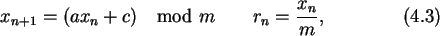where m, a, and c are three parameters which determine the quality of the generated numbers. Clearly the maximum period of the pseudo-random number sequence is m. Thus m will usually be chosen close to the maximum integer number representable in a single register of the processor. The other two parameters a and c should be relatively prime to m.

The linear congruential method has the advantage of being very fast, requiring only a few operations per call. It has the disadvantage that it is not free of sequential correlation on successive calls. Thus for SIMON we are using a random generator that      shuffles the output of a linear congruential method to remove the least significant bit correlations . To achieve the shuffling, a computed random number rn is not output as the n-th random number, but rather at a randomized later call.

Another derivative of a linear congruential method is a    nonlinear congruential method, of which the inversive congruential method is a special case.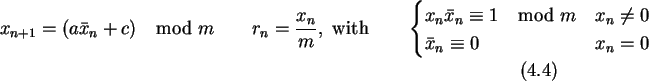The    inversive congruential method has superior statistical properties compared to the plain linear congruential method . On the other hand the calculation of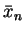for a given xn consumes additional computational resources and thus this method is not so efficient. The Euclidean algorithm to calculatefor a given xn from  terminates in average after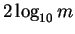steps.

The    shift-register generators received their name from the fact, that such generators can be built in hardware from shift registers with a feedback. If m is a small prime, usually m=2, a shift-register method can be written aswith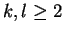and integers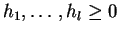. In the case of m=2shift-register generators can be implemented efficiently since only binary arithmetic is needed. They too have better statistical properties than a simple linear congruential method.

A complicated computation of random numbers does not necessarily guarantee their randomness. In the contrary the more complicate the harder it is to derive theoretical justifications for the soundness of the method. However, the only way to guarantee the quality of a random generator is thorough testing. One test for the accuracy of the probability distribution of the PRN is to plot their probability function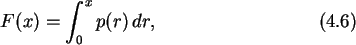where p(r) is the probability distribution of the PRN. The function F(x) gives the probability that the random number r is smaller than x. Fig. 4.6 compares F(x) for x<10-4 for the above explained random generator that SIMON uses and a simple linear congruential method with the parameters (m,a,c)=(714025,1366,150889).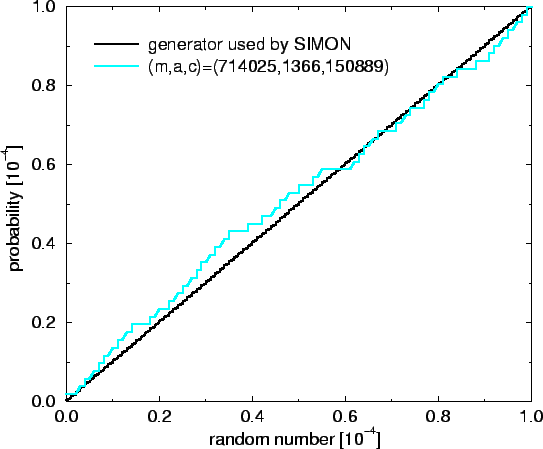The simple linear congruential method shows deviations to the ideal characteristic F(x)=x, and bigger steps in the fine structure. Fig. 4.6 shows only the interval [0,10-4], however, a similar behavior is found in the remaining part [10-4,1]. The  lattice structure is another important property of PRN-generators . The presence of a regular lattice structure can be assessed by looking at points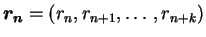. In the case of linear congruential methods all rn lie on relatively few parallel hyperplanes, as is shown on the left side of Fig. 4.7.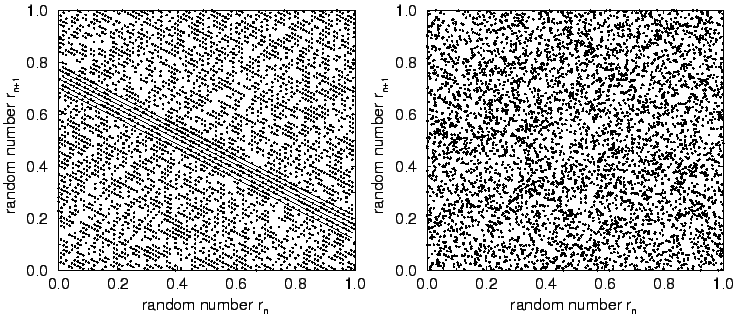In comparison the right side shows points for the shuffled congruential method used in SIMON. There such parallel hyperplanes are not visible.Next: Step Size Up: 4.2 Code structure Previous: 4.2.2 Flow Chart

Christoph Wasshuber